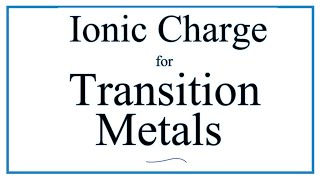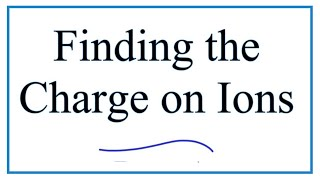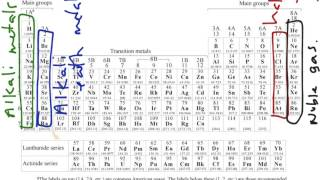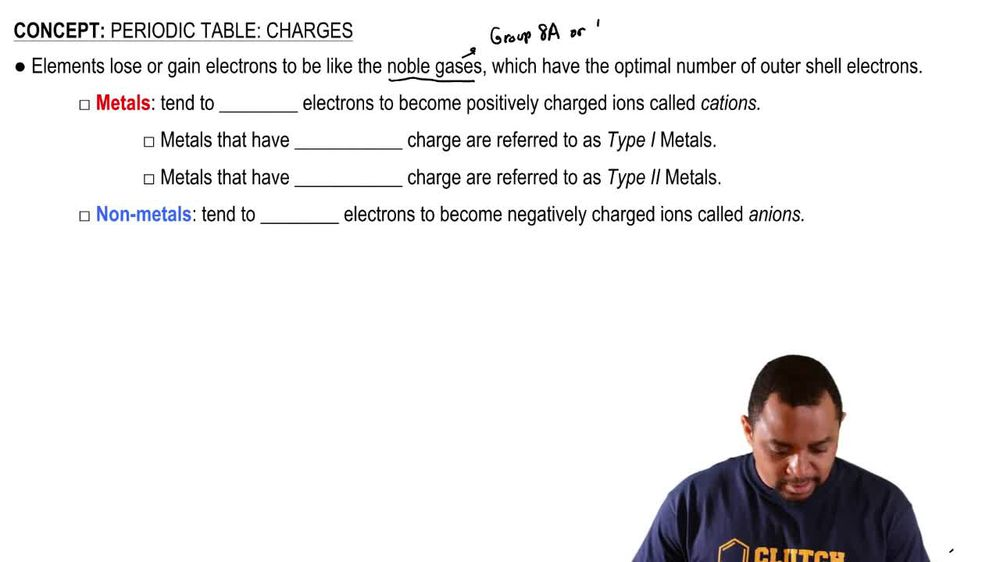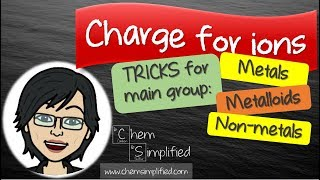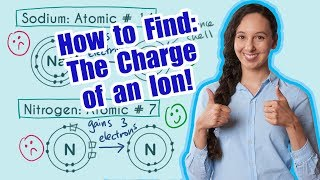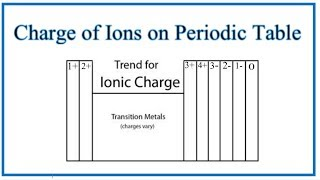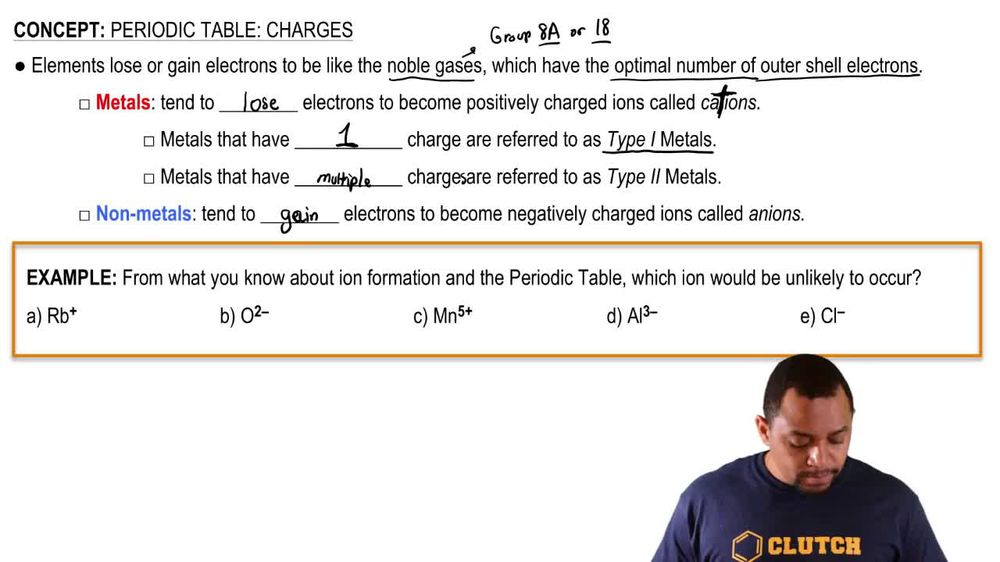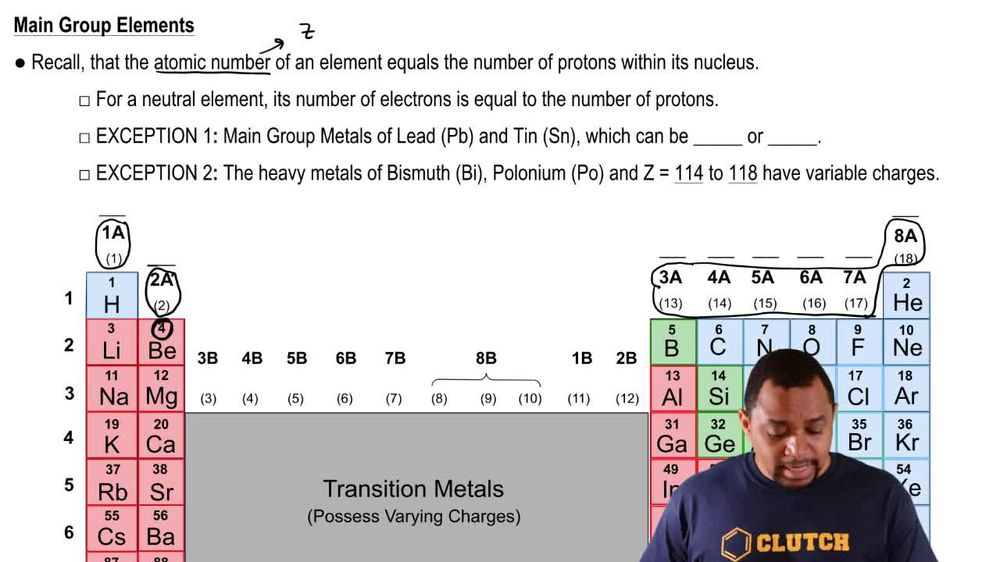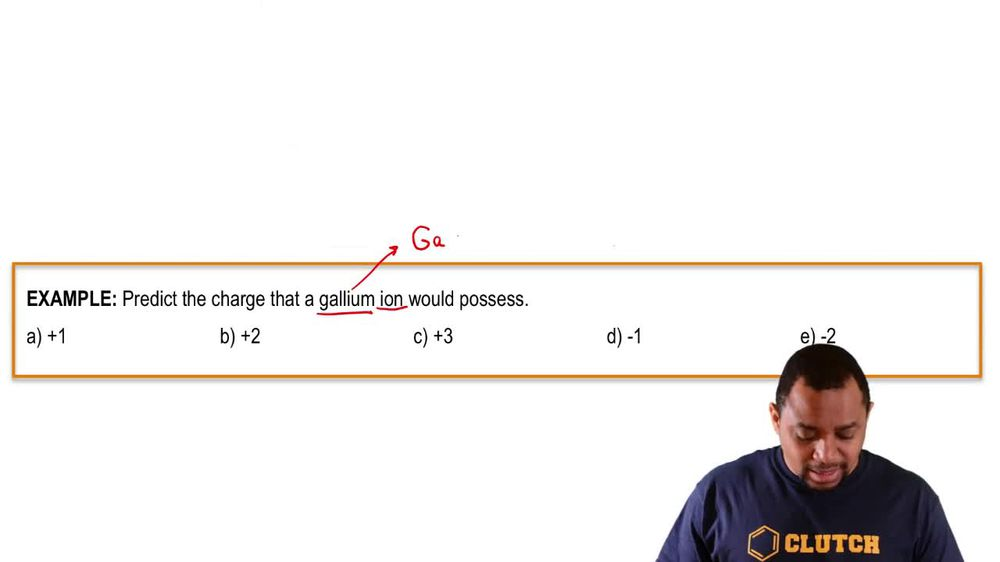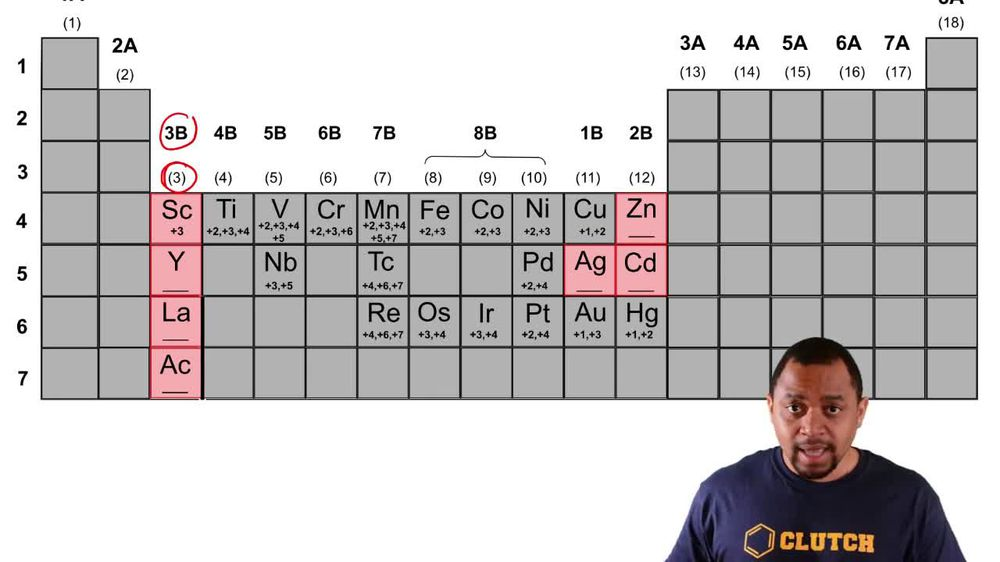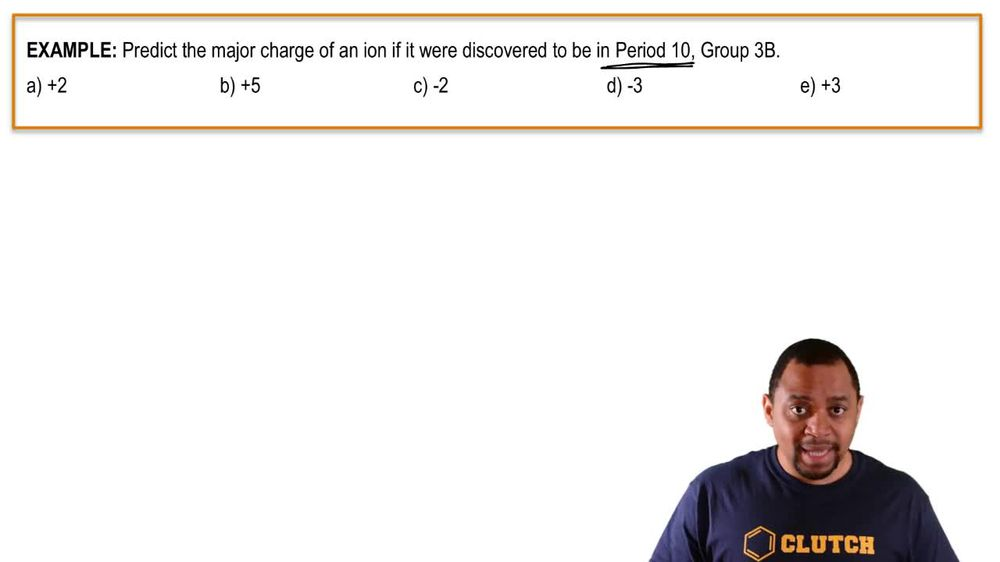Start typing, then use the up and down arrows to select an option from the list.
1. 2. Atoms & Elements2. Periodic Table: Charges# Periodic Table: Charges Example 1

by Jules Bruno
132 views
1
1
So for this example question. It says, from what you know about ion formation in the periodic table, which ion would be unlikely to occur. So before we look at this question, remember, we're gonna say that medals, they tend to lose electrons, so they're gonna lose electrons. They're going to become positively charged. And remember, non metals tend to gain electrons and therefore becoming negatively charged. So if we take a look here, we have our be looking on a periodic table. We know that our B has is a metal and therefore it makes sense that it would have a positive charge. So this is a likely set up for R B I on next oxygen o oxygen is a non metal nonmetal tend to gain electrons have become negatively charged. So this is reasonable. M N m n is a metal again. Medals tend to lose electrons to become positively charged, so that positive charge is a possibility. A l A. L is aluminum. Aluminum is a metal again. Medals tend to lose electrons to become positively charged, so it doesn't make sense that l would have a negative three charge here, So this is unlikely. So This would be our answer then, if we look at the last one. Cl chlorine. Chlorine is a non metal, so it makes sense it could gain electrons to become negatively charged. So just remember, remember, metals tend to lose electrons to become positively charged. Non metals tend to gain electrons to become negatively charged. Okay, now that we know this fundamental idea, let's move on to our next video. Where we go into, how many electrons do they gain? How many electrons do they lose and in that way, know what type of ions are possible for certain types of elements.